# Math in Focus Grade 5 Chapter 10 Practice 1 Answer Key Percent

Practice the problems of Math in Focus Grade 5 Workbook Answer Key Chapter 10 Practice 1 Percent to score better marks in the exam.

## Math in Focus Grade 5 Chapter 10 Practice 1 Answer Key Percent

Each large square is divided into 100 parts. Fill in the blanks to describe each large square.

Question 1.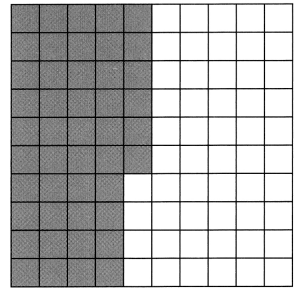___ out of 100 equal parts are shaded.
___ % of the large square is shaded.
___ out of 100 equal parts are not shaded.
____ % of the large square is not shaded.
46 out of 100 equal parts are shaded.
46 % of the large square is shaded.
54 out of 100 equal parts are not shaded.
54 % of the large square is not shaded.
Explanation:
Each large scale is divided into 100 squares,
So the percentage of large scale are 46%  and 54%

Question 2.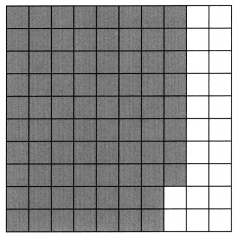___ out of 100 equal parts are shaded.
___ % of the large square is shaded.
___ out of 100 equal parts are not shaded.
____ % of the large square is not shaded.
78 out of 100 equal parts are shaded.
78 % of the large square is shaded.
22 out of 100 equal parts are not shaded.
22 % of the large square is not shaded.
Explanation:
Each large scale is divided into 100 squares,
So the percentage of large scale are 78%  and 22%

Express each decimal as a percent

Example
$$\frac{38}{100}$$ = 38%

Question 3.
$$\frac{7}{100}$$ = ___ %
7%
Explanation:
To convert a fraction to a percent,
first divide the numerator by the denominator.
Then multiply the decimal by 100.
The fraction $$\frac{7}{100}$$ can be converted to decimal by dividing 7 by 100.
It can be converted to percent by multiplying the decimal by 100.
7 ÷ 100 = 0.07×100 = 7
So, the fraction $$\frac{7}{100}$$ is equivalent to 7%

Question 4.
$$\frac{7}{100}$$ = ___ %
7%
Explanation:
To convert a fraction to a percent,
first divide the numerator by the denominator.
Then multiply the decimal by 100.
The fraction $$\frac{7}{100}$$ can be converted to decimal by dividing 7 by 100.
It can be converted to percent by multiplying the decimal by 100.
7 ÷ 100 = 0.07×100 = 7
So, the fraction $$\frac{7}{100}$$ is equivalent to 7%

Question 5.
$$\frac{19}{100}$$ = ___ %
19%
Explanation:
To convert a fraction to a percent,
first divide the numerator by the denominator.
Then multiply the decimal by 100.
The fraction $$\frac{19}{100}$$ can be converted to decimal by dividing 19 by 100.
It can be converted to percent by multiplying the decimal by 100.
19 ÷ 100 = 0.19×100 = 19
So, the fraction $$\frac{19}{100}$$ is equivalent to 19%

Question 6.
$$\frac{6}{10}$$ = ___ %
60%
Explanation:
To convert a fraction to a percent,
first divide the numerator by the denominator.
Then multiply the decimal by 100.
The fraction $$\frac{6}{10}$$ can be converted to decimal by dividing 6 by 10.
It can be converted to percent by multiplying the decimal by 100.
6 ÷ 10 = 0.6×100 = 60
So, the fraction $$\frac{6}{10}$$ is equivalent to 60%

Question 7.
$$\frac{4}{10}$$ = ___ %
40%
Explanation:
To convert a fraction to a percent,
first divide the numerator by the denominator.
Then multiply the decimal by 100.
The fraction $$\frac{4}{10}$$ can be converted to decimal by dividing 4 by 10.
It can be converted to percent by multiplying the decimal by 100.
4 ÷ 10 = 0.4×100 = 40
So, the fraction $$\frac{4}{10}$$ is equivalent to 40%

Express each decimal as a percent.

Example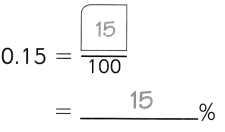Question 8.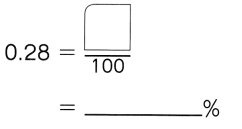Explanation:
To convert a decimal to a fraction, place the decimal number over its place value.
First divide the numerator by the denominator.
Then multiply the decimal by 100, to convert a fraction to a percent.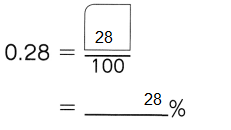Question 9.
0.07 = _________%
Explanation:
To convert a decimal to a fraction, place the decimal number over its place value.
First divide the numerator by the denominator.
Then multiply the decimal by 100, to convert a fraction to a percent.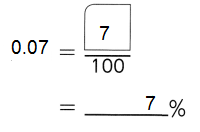Question 10.
0.01 = _________%
Explanation:
To convert a decimal to a fraction, place the decimal number over its place value.
First divide the numerator by the denominator.
Then multiply the decimal by 100, to convert a fraction to a percent.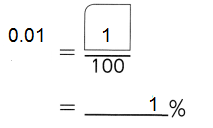Question 11.
0.08 _________%
Explanation:
To convert a decimal to a fraction, place the decimal number over its place value.
First divide the numerator by the denominator.
Then multiply the decimal by 100, to convert a fraction to a percent.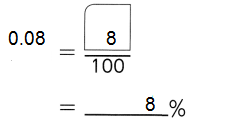Question 12.
0.5 = _________%
Explanation:
To convert a decimal to a fraction, place the decimal number over its place value.
First divide the numerator by the denominator.
Then multiply the decimal by 100, to convert a fraction to a percent.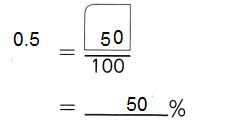Question 13.
0.9 = _________%
Explanation:
To convert a decimal to a fraction, place the decimal number over its place value.
First divide the numerator by the denominator.
Then multiply the decimal by 100, to convert a fraction to a percent.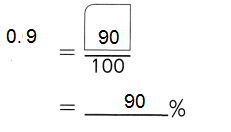Question 14.
0.8 = _________%
Explanation:
To convert a decimal to a fraction, place the decimal number over its place value.
First divide the numerator by the denominator.
Then multiply the decimal by 100, to convert a fraction to a percent.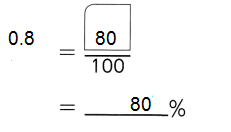Express each percent as a fraction with a denominator of 100.

Example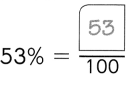Question 15.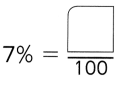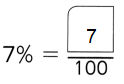Explanation:
To convert a percent to a fraction,
we have to remove the percent sign and divide the given number by 100.

Question 16.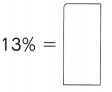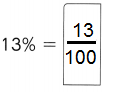Explanation:
To convert a percent to a fraction,
we have to remove the percent sign and divide the given number by 100.

Question 17.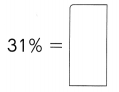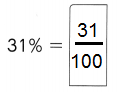Explanation:
To convert a percent to a fraction,
we have to remove the percent sign and divide the given number by 100.

Question 18.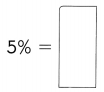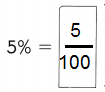Explanation:
To convert a percent to a fraction,
we have to remove the percent sign and divide the given number by 100.

Question 19.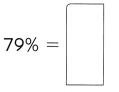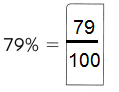Explanation:
To convert a percent to a fraction,
we have to remove the percent sign and divide the given number by 100.

Express each percent as a fraction with a denominator of 100.

Example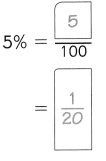Question 20.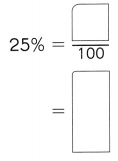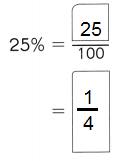Explanation:
To convert a percent to a fraction,
we have to remove the percent sign and divide the given number by 100.
And, then we express the fractional form of the percentage in the simplest form.

Question 21.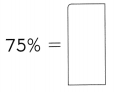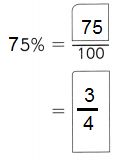Explanation:
To convert a percent to a fraction,
we have to remove the percent sign and divide the given number by 100.
And, then we express the fractional form of the percentage in the simplest form.

Question 22.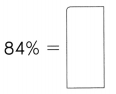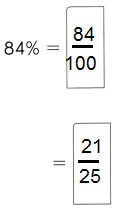Explanation:
To convert a percent to a fraction,
we have to remove the percent sign and divide the given number by 100.
And, then we express the fractional form of the percentage in the simplest form.

Question 23.
46% =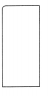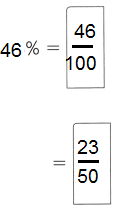Explanation:
To convert a percent to a fraction,
we have to remove the percent sign and divide the given number by 100.
And, then we express the fractional form of the percentage in the simplest form.

Question 24.
55% =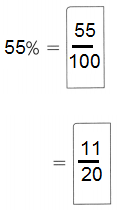Explanation:
To convert a percent to a fraction,
we have to remove the percent sign and divide the given number by 100.
And, then we express the fractional form of the percentage in the simplest form.

Express each percent as a decimal.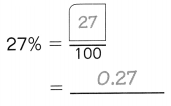Question 25.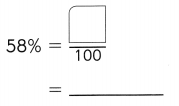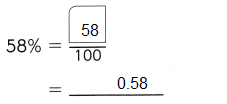Explanation:
First remove the percent sign to convert from a percent to a decimal.
Divide the number by 100 and the out put is in the form of decimal.

Question 26.
9% = ___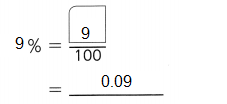Explanation:
First remove the percent sign to convert from a percent to a decimal.
Divide the number by 100 and the out put is in the form of decimal.

Question 27.
1% = ___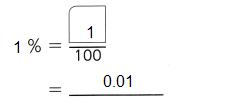Explanation:
First remove the percent sign to convert from a percent to a decimal.
Divide the number by 100 and the out put is in the form of decimal.

Write each ratio as a fraction and then as a percent.

Question 28.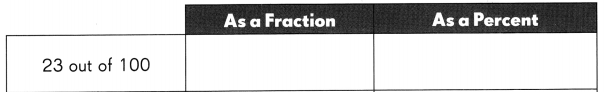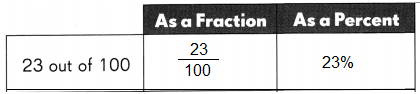Explanation:
Ratios are smaller to fractions, and each fraction can be written as a fraction.
Divide the first number 23 by the second number 100.
Multiply by 100 to convert to a percentage.
Add the percent symbol (%) to the output.

Question 29.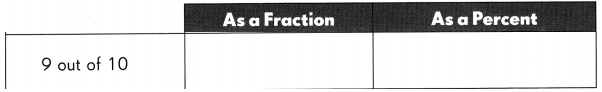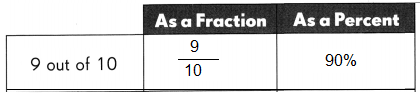Explanation:
Ratios are smaller to fractions, and each fraction can be written as a fraction.
Divide the first number 9 by the second number 100.
Multiply by 100 to convert to a percentage.
Add the percent symbol (%) to the output

Express each percent as a decimal. Then mark ✗ to show where each decimal is located on the number line.

Question 30.
71% = ______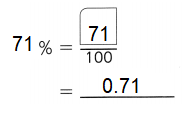Explanation:
To convert a decimal to a percentage, multiply by 100,
just move the decimal point 2 places to the right.

Question 31.
19% = ___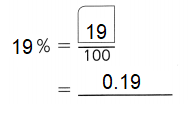Explanation:
To convert a decimal to a percentage, multiply by 100,
just move the decimal point 2 places to the right.

Question 32.
44%= ____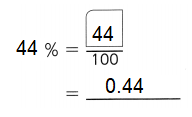Explanation:
To convert a decimal to a percentage, multiply by 100,
just move the decimal point 2 places to the right.

Question 33.
There are 100 students in a drawing contest, and 58 of them are girls.
a. What percent of the students in the contest are girls?
Explanation:
To convert a fraction to a percent,
first divide the numerator by the denominator.
Then multiply the decimal by 100.
The fraction $$\frac{58}{100}$$ can be converted to decimal by dividing 58 by 100.
It can be converted to percent by multiplying the decimal by 100.
58 ÷ 100 = 0.58×100 = 58
So, the fraction $$\frac{58}{100}$$ is equivalent to 58%

b. What percent of the students in the contest are boys?
Explanation:
To convert a fraction to a percent,
first divide the numerator by the denominator.
Then multiply the decimal by 100.
The fraction $$\frac{42}{100}$$ can be converted to decimal by dividing 42 by 100.
It can be converted to percent by multiplying the decimal by 100.
42 ÷ 100 = 0.42×100 = 42
So, the fraction $$\frac{42}{100}$$ is equivalent to 42%

Question 34.
A jogging route is 10 kilometers long. Lee Ming has jogged 4 kilometers of the route.

a. What percent of the route has Lee Ming jogged?
Explanation:
A jogging route is 10 kilometers long.
Lee Ming has jogged 4 kilometers of the route.
To convert a fraction to a percent,
first divide the numerator by the denominator.
Then multiply the decimal by 100.
The fraction $$\frac{4}{10}$$ can be converted to decimal by dividing 4 by 10.
It can be converted to percent by multiplying the decimal by 100.
4 ÷ 10 = 0.4×100 = 40
So, the fraction $$\frac{4}{10}$$ is equivalent to 40%

b. What percent of the route does Lee Ming have to jog to complete the whole route?
The fraction $$\frac{6}{10}$$ can be converted to decimal by dividing 6 by 10.
So, the fraction $$\frac{6}{10}$$ is equivalent to 60%